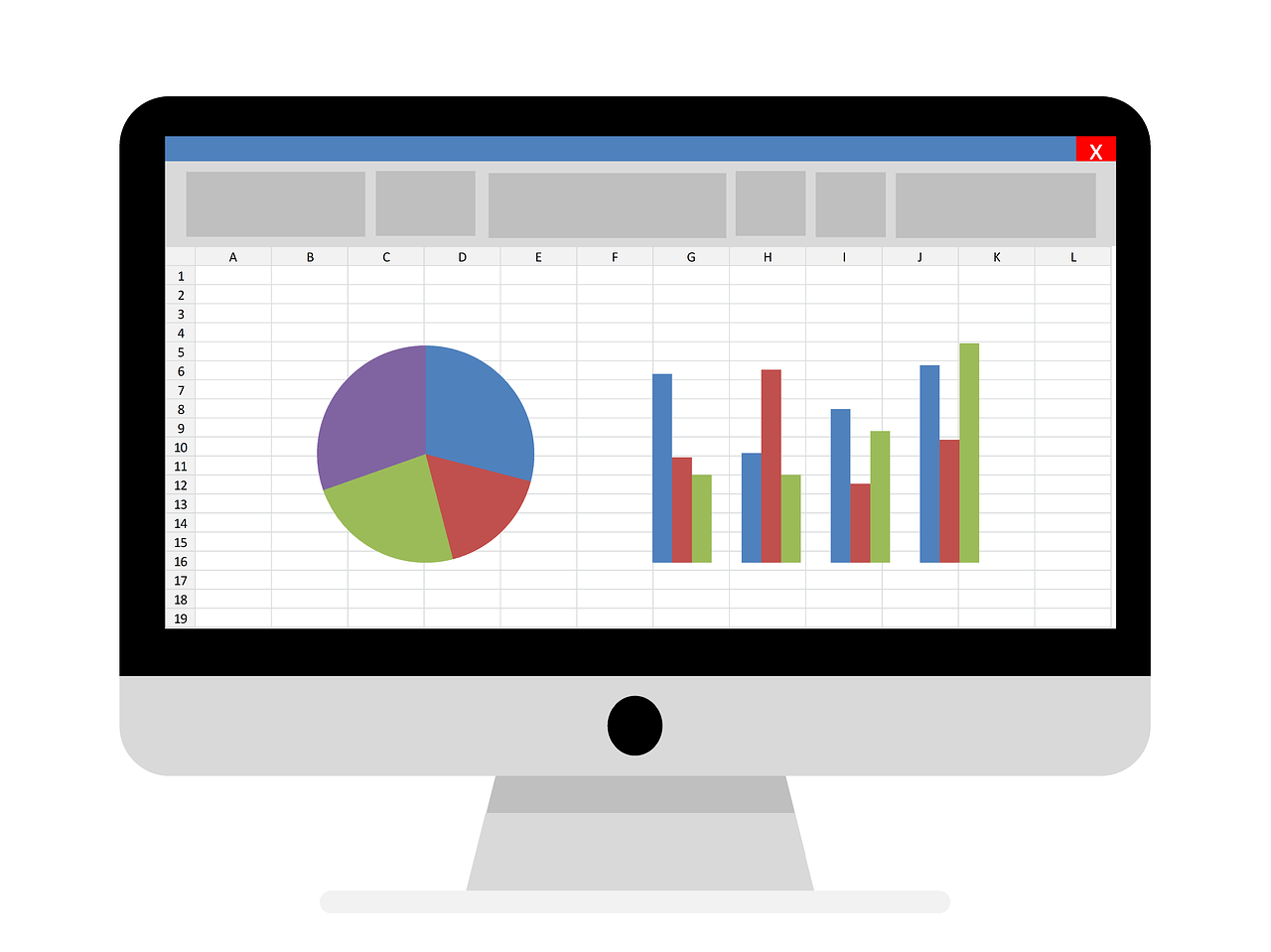Office tutorials

Latest Articles

VBA For Next Loop

For Next VBA loop – basic information The For Next VBA loop is another loop that we will meet. In the […]

VBA Do While Loop and Do Until Loop

The diagram of Do While Loop The While loop … Wend , which we met in the previous chapter is the simplest loop in […]

VBA While Wend Loop

While Wend loop – introduction The While Wend loop is the simplest in its construction loop available in VBA. If our […]

Excel VBA Loops – Introduction

Introduction to the loops in Excel VBA Loops are the strongest element of programming languages. It is through them that we […]Select CASE statement in Excel VBA

SELECT CASE instruction – introduction Another instruction next to IF THEN ELSE used in the decision making process by our program is […]IF THEN ELSE statement in Excel VBA

What is the conditional statement If Then Else VBA In the simplest sense, this is the equivalent of the ” If […]Worksheet functions in Excel VBA

Worksheet functions – How to use the Excel function in VBA  Have you ever wondered how we can use the […]Creating functions in Excel VBA – User functions

Creating own function defined by user Both Excel and VBA offer us a number of useful functions. But what if we […]Date and time functions in Excel VBA

Description of date and time functions. The VBA date functions as per the name indicate are used to manipulate values […]VBA character functions

VBA character functions in Excel The Excel VBA offers us a number of useful character functions. We can not compare them […]